Nota

Lección desarrollada por Linfiniti y S Motala (Universidad Tecnológica de Península del Cabo)

Spatial statistics allows you to analyze and understand what is going on in a given vector dataset. QGIS includes many useful tools for statistical analysis.

The goal for this lesson: To know how to use QGIS’ spatial statistics tools within the Processing Toolbox.

## 6.4.1.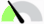Follow Along: Crear un Conjunto de Datos de Prueba¶

We will create a random set of points, to get a dataset to work with.

To do so, you will need a polygon dataset to define the area you want to create the points in.

We will use the area covered by streets.

1. Start a new project

2. Add your `roads` dataset, as well as `srtm_41_19` (elevation data) found in `exercise_data/raster/SRTM/`.

Nota

You might find that the SRTM DEM layer has a different CRS to that of the roads layer. QGIS is reprojecting both layers in a single CRS. For the following exercises this difference does not matter, but feel free to reproject (as shown earlier in this module).

3. Open Processing toolbox

4. Use the Vector Geometry ▶ Minimum bounding geometry tool to generate an area enclosing all the roads by selecting `Convex Hull` as the Geometry Type: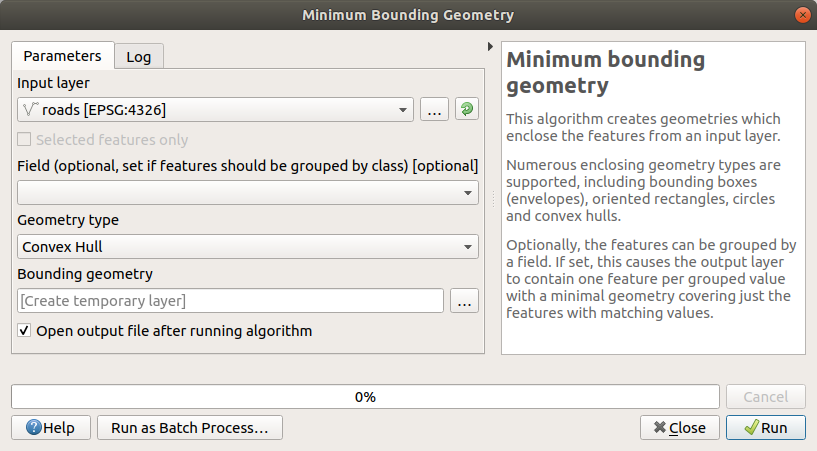As you know, if you don’t specify the output, Processing creates temporary layers. It is up to you to save the layers immediately or at a later stage.

### 6.4.1.1. Creación de puntos al azar¶

• Create 100 random points in this area using the tool at Vector Creation ▶ Random points in layer bounds, with a minimum distance of `0.0`: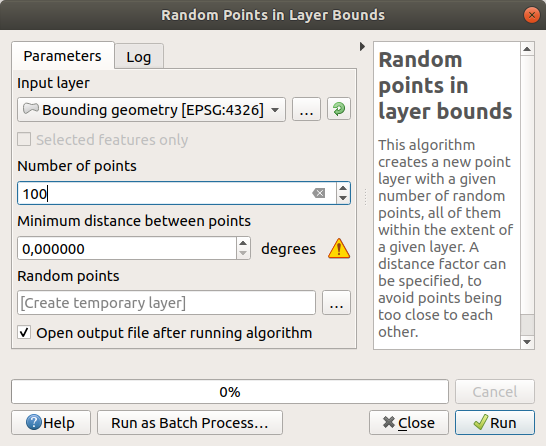Nota

The yellow warning sign tells you that that parameter concerns distances. The Bounding geometry layer is in a Geographical Coordinate System and the algorithm is just reminding you this. For this example we won’t use this parameter so you can ignore it.

If needed, move the generated random point to the top of the legend to see them better: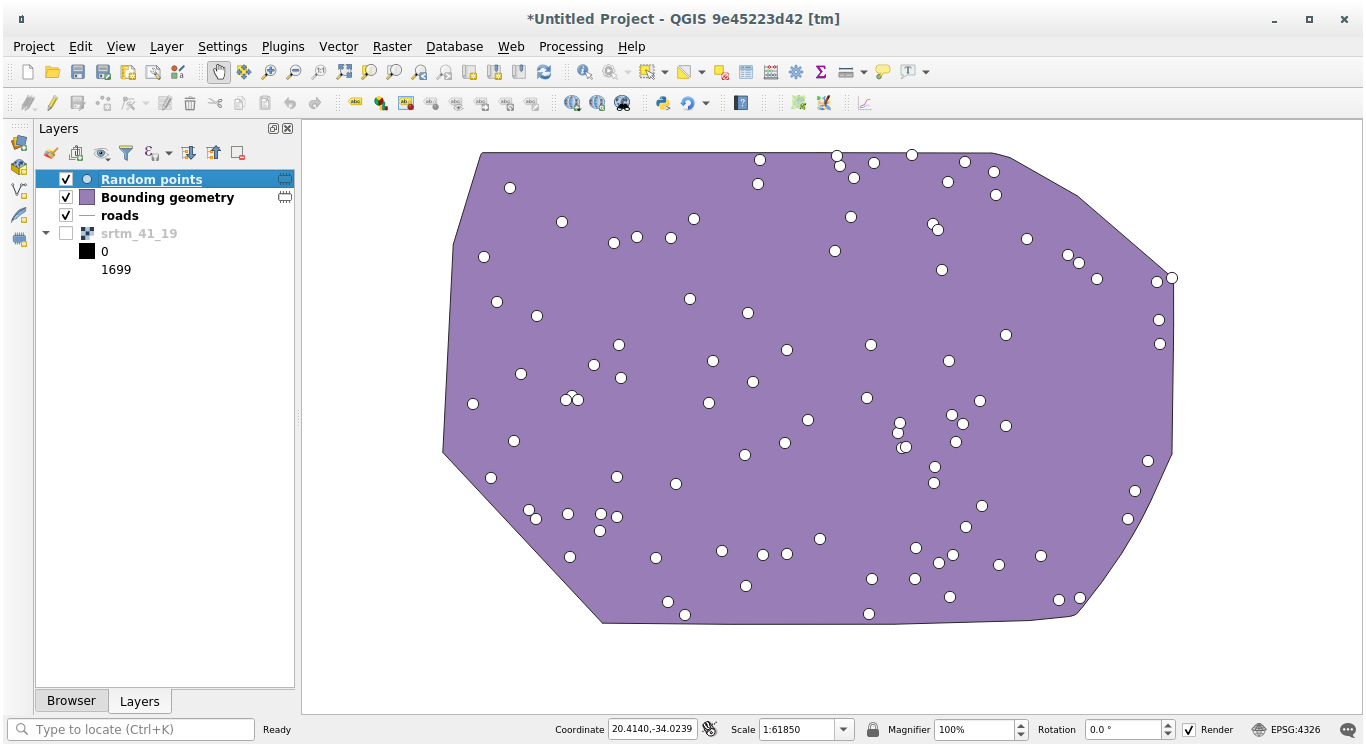### 6.4.1.2. Muestreo de los datos¶

To create a sample dataset from the raster, you’ll need to use the Raster Analysis ▶ Sample raster values algorithm. This tool samples the raster at the locations of the points and adds the raster values in new field(s) depending on the number of bands in the raster.

1. Abra a caixa de diálogo do algoritmo Amostrar valores do raster

2. Select `Random_points` as the layer containing sampling points, and the SRTM raster as the band to get values from. The default name of the new field is `rvalue_N`, where `N` is the number of the raster band. You can change the name of the prefix if you want.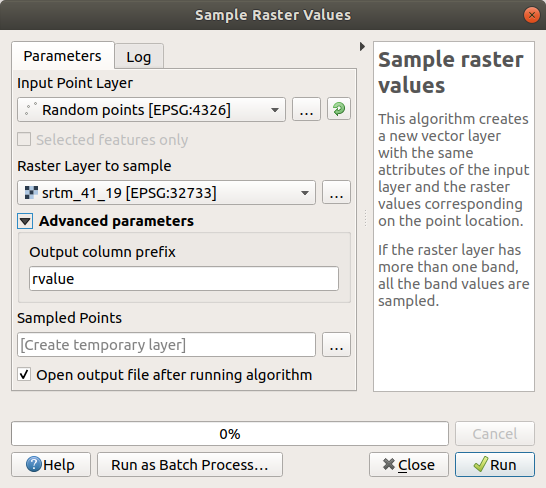3. Pressione Executar

Now you can check the sampled data from the raster file in the attribute table of the `Sampled Points` layer. They will be in a new field with the name you have chosen.

Aquí tienes una posible capa de muestreo: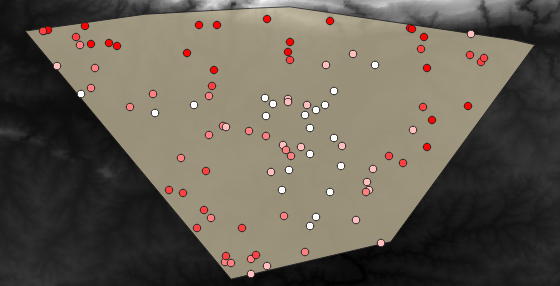The sample points are classified using the `rvalue_1` field such that red points are at a higher altitude.

You will be using this sample layer for the rest of the statistical exercises.

## 6.4.2.Follow Along: Estadísticas Básicas¶

Ahora obtén lsa estadísticas básica de esta capa.

1. Click on the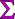Show statistical summary icon in the Attributes Toolbar. A new panel will pop up.

2. In the dialog that appears, specify the `Sampled Points` layer as the source.

3. Select the rvalue_1 field in the field combo box. This is the field you will calculate statistics for.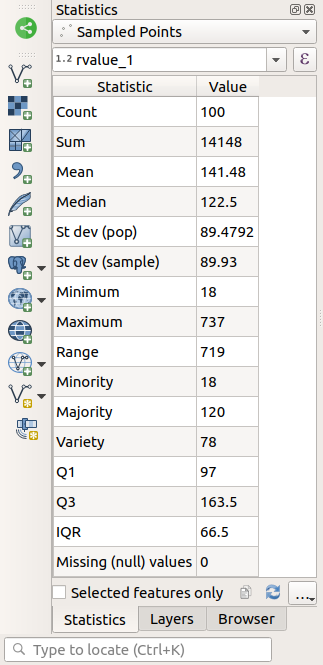Nota

You can copy the values by clicking on the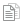Copy Statistics To Clipboard button and paste the results into a spreadsheet.

5. Close the Statistics Panel when done

Many different statistics are available:

Contagem

The number of samples/values.

Suma

Media

The mean (average) value is simply the sum of the values divided by the number of values.

Mediana

If you arrange all the values from smallest to greatest, the middle value (or the average of the two middle values, if N is an even number) is the median of the values.

St Dev (pop)

La desviación estándar. Da una indicación de cómo de cerca se agrupan los valores alrededor de la media. Cuanto menor sea la desviación estándar, más cerca estarán los valores a la media.

Mĩnimo

El valor mínimo

Máximo

El valor máximo.

Intervalo

La diferencia entre los valores mínimo y máximo.

Q1

Q3

Missing (null) values

The number of missing values.

## 6.4.3.Follow Along: Compute statistics on distances between points¶

1. Create a new temporary point layer.

2. Enter edit mode, and digitize three points somewhere among the other points.

Como alternativa, use o mesmo método de geração de pontos aleatórios de antes, mas especifique apenas três pontos.

3. Salve sua nova camada como distance_points no formato que você preferir.

Para gerar estatísticas sobre as distâncias entre pontos nas duas camadas:

1. Open the Vector Analysis ▶ Distance matrix tool.

2. Select the `distance_points` layer as the input layer, and the `Sampled Points` layer as the target layer.

3. Ajústalo así: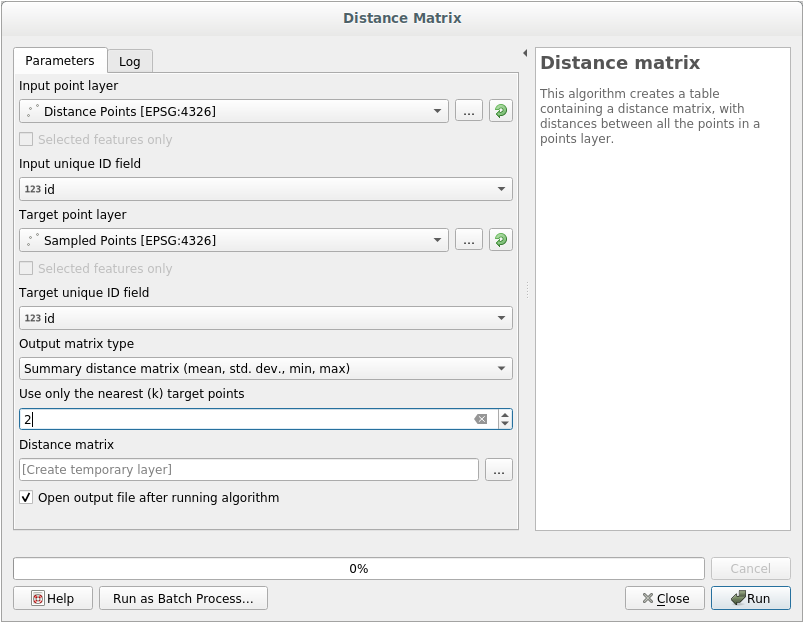4. If you want you can save the output layer as a file or just run the algorithm and save the temporary output layer later.

5. Clique em Executar para gerar a camada da matriz de distância.

6. Abra a tabela de atributos da camada gerada: valores referem-se às distâncias entre as feições distance_points e seus dois pontos mais próximos na camada Pontos de amostra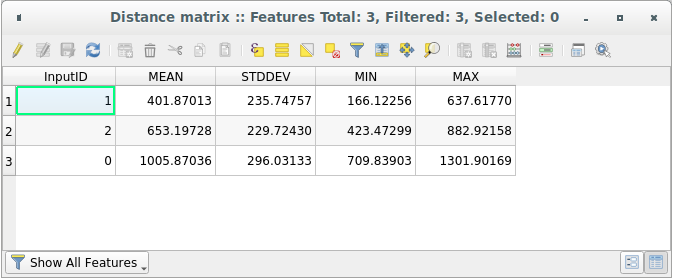With these parameters, the Distance Matrix tool calculates distance statistics for each point of the input layer with respect to the nearest points of the target layer. The fields of the output layer contain the mean, standard deviation, minimum and maximum for the distances to the nearest neighbors of the points in the input layer.

## 6.4.4.Follow Along: Análise de Vizinhos mais Próximos (dentro da camada)¶

Para fazer uma análise de vizinho mais próximo de uma camada de pontos:

1. Choose Vector analysis ▶ Nearest neighbor analysis.

2. Na caixa de diálogo exibida, selecione a camada Pontos aleatórios e clique em Executar.

3. The results will appear in the Processing Result Viewer Panel.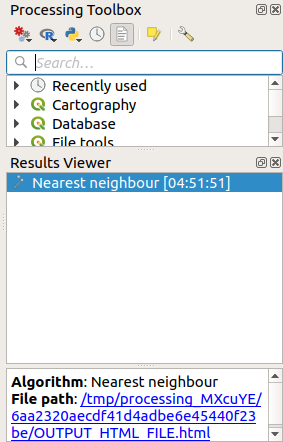4. Clique no link azul para abrir a página `html` com os resultados: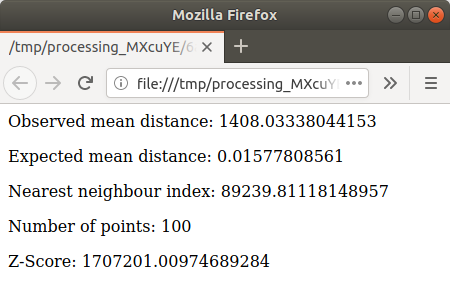## 6.4.5.Follow Along: Coordenadas Medias¶

Para obtener las coordenadas medias de un conjunto de datos:

1. Start Vector analysis ▶ Mean coordinate(s)

2. In the dialog that appears, specify Random points as Input layer, and leave the optional choices unchanged.

3. Haz clic en Run.

Let us compare this to the central coordinate of the polygon that was used to create the random sample.

1. Start Vector geometry ▶ Centroids

2. In the dialog that appears, select `Bounding geometry` as the input layer.

As you can see, the mean coordinates (pink point) and the center of the study area (in green) don’t necessarily coincide.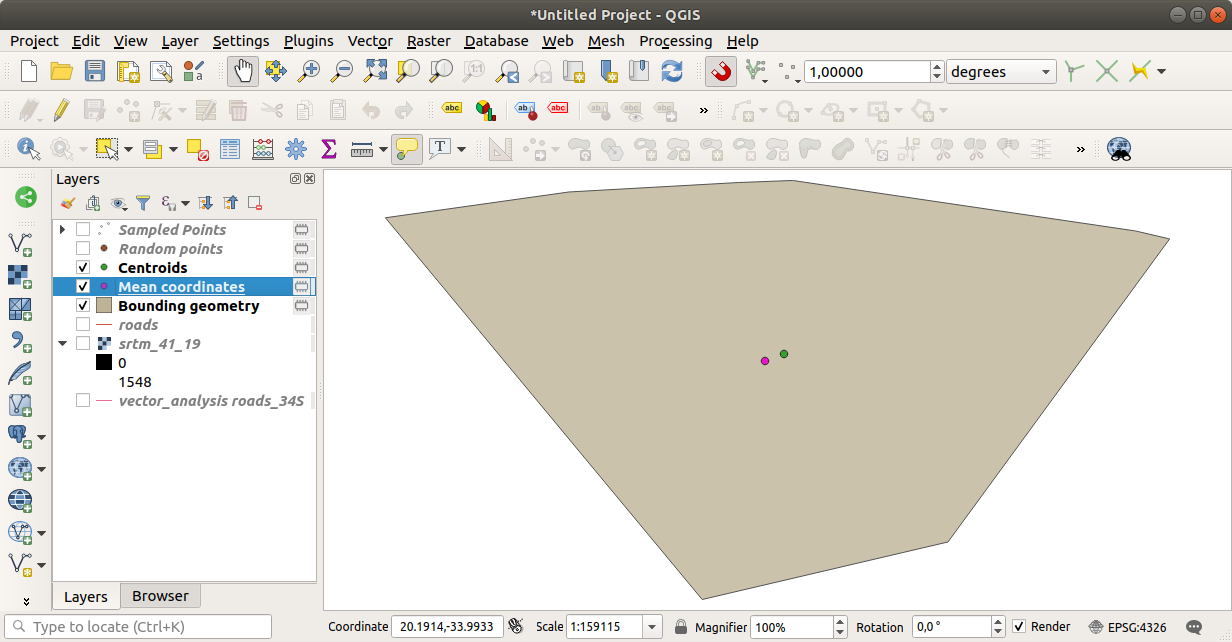## 6.4.6.Follow Along: Histogramas de Imagenes¶

O histograma de um conjunto de dados mostra a distribuição de seus valores. A maneira mais simples de demonstrar isso no QGIS é através do histograma da imagem, disponível na caixa de diálogo Propriedades da Camada de qualquer camada da imagem (conjunto de dados raster).

1. In your Layers panel, right-click on the `srtm_41_19` layer

2. Select Properties

3. Choose the Histogram tab. You may need to click on the Compute Histogram button to generate the graphic. You will see a graph that shows the frequency distribution for the raster values.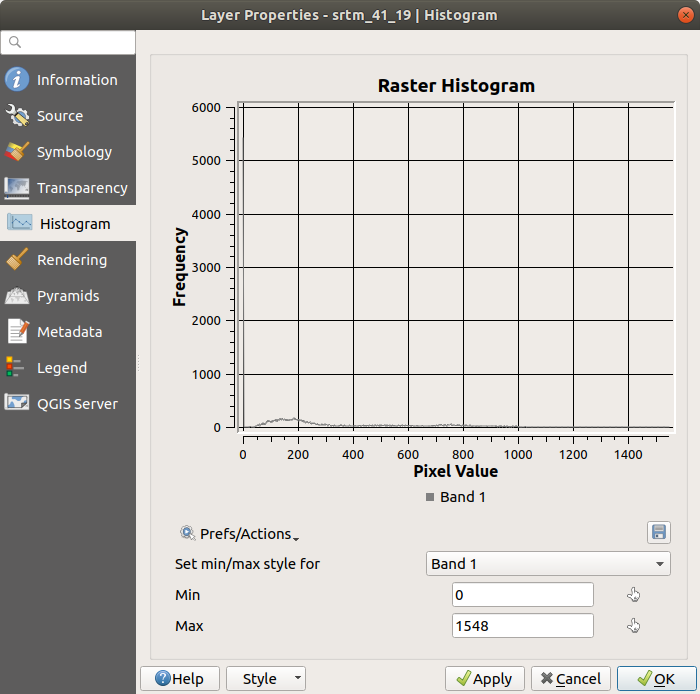4. The graph can be exported as an image with the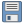Save plot button

5. You can see more detailed information about the layer in the Information tab (the mean and max values are estimated, and may not be exact).

The mean value is `332.8` (estimated to 324.3), and the maximum value is `1699` (estimated to 1548)! You can zoom in the histogram. Since there are a lot of pixels with value `0`, the histogram looks compressed vertically. By zooming in to cover everything but the peak at `0`, you will see more details: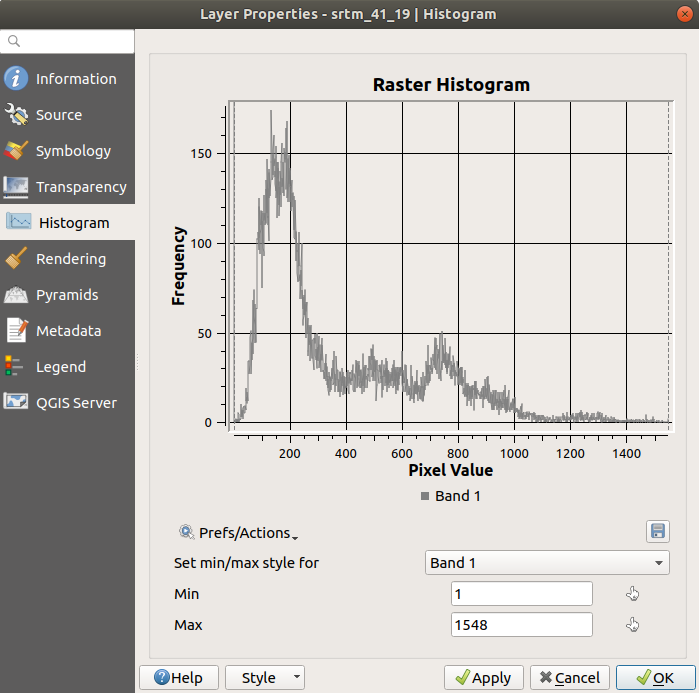Nota

If the mean and maximum values are not the same as above, it can be due to the min/max value calculation. Open the Symbology tab and expand the Min / Max Value Settings menu. Choose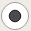Min / max and click on Apply.

Keep in mind that a histogram shows you the distribution of values, and not all values are necessarily visible on the graph.

## 6.4.7.Follow Along: Interpolación Espacial¶

Let’s say you have a collection of sample points from which you would like to extrapolate data. For example, you might have access to the Sampled points dataset we created earlier, and would like to have some idea of what the terrain looks like.

1. To start, launch the GDAL ▶ Raster analysis ▶ Grid (IDW with nearest neighbor searching) tool in the Processing Toolbox.

2. For Point layer select `Sampled points`

3. Set Weighting power to `5.0`

4. In Advanced parameters, set Z value from field to `rvalue_1`

5. Finally click on Run and wait until the processing ends

6. Feche a caixa de diãlogo

Here is a comparison of the original dataset (left) to the one constructed from our sample points (right). Yours may look different due to the random nature of the location of the sample points.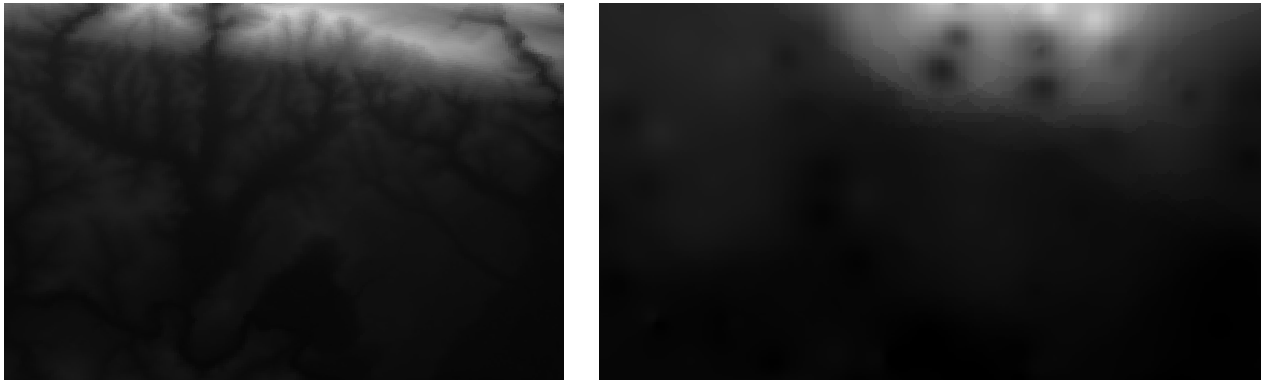Como você pode ver, 100 pontos de amostra não são suficientes para obter uma impressão detalhada do terreno. Dá uma ideia muito geral, mas também pode ser enganosa.

## 6.4.8.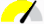Try Yourself Mẽtodos de interpolação diferentes¶

1. Use the processes shown above to create a set of 10 000 random points

Nota

If the number of points is really big, the processing time can take a long time.

2. Use these points to sample the original DEM

3. Use the Grid (IDW with nearest neighbor searching) tool on this dataset.

4. Set Power and Smoothing to `5.0` and `2.0`, respectively.

Los resultados (dependiendo de la posición de tus puntos aleatorios) se verán más o menos como esto: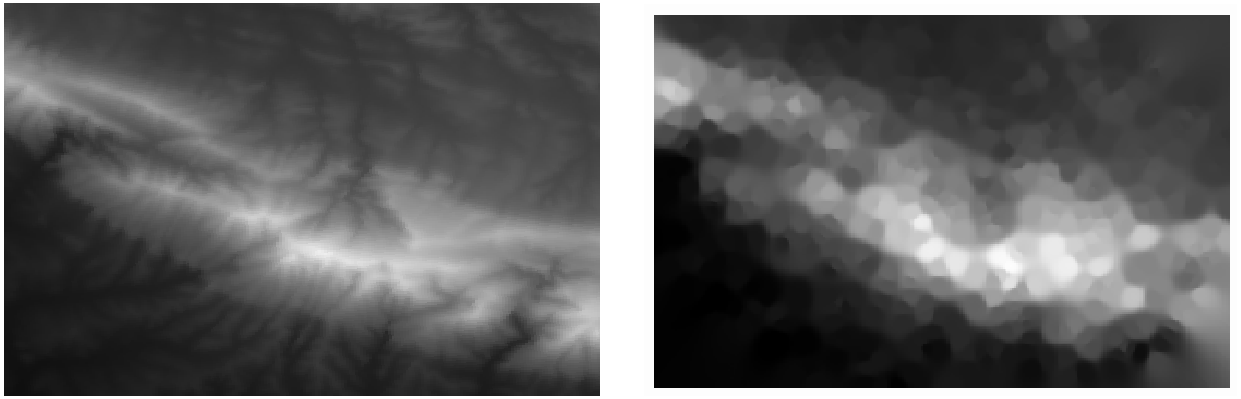This is a better representation of the terrain, due to the greater density of sample points. Remember, larger samples give better results.

## 6.4.9. In Conclusion¶

QGIS has a number of tools for analyzing the spatial statistical properties of datasets.

## 6.4.10. What’s Next?¶

Now that we have covered vector analysis, why not see what can be done with rasters? That is what we will do in the next module!